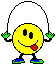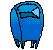Elementary Algebra Assignment #4C Solve for x :1. x + 5 = 10 2. 5x = 10 3. x - 3 = 18 4. -3x = 18 5. x + 5 = -15 6. 5x = -15 7. x - 2 = -8 8. -2x = -8 9. -8 + x = 24 10. 24 = -8x 11. -7 = x + 5 12. -80 = 2x 13. 12 = -2 + xemailBACK TO HOME PAGE